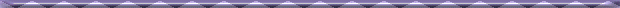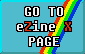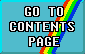# GETTING SORTED

### by Tim Hartnell Your Sinclair - April 1987Did you know that 90 percent of all computer programs do some kind of sorting? Well, they do according to Jonathan Amsterdam (Byte Magazine Sept '85), and that's a whole lot of programs.

The most basic type of sort is where a series of strings are placed in alphabetical order or a list of numbers are put in ascending or descending order. Whether you're sorting names of products in a storeroom, a mail list ranked in the Post Office's peculiar number and letter combination or the results, from highest to lowest within a class, of an examination, similar sorting techniques can be used.

However there's a mind-boggling number of sorting algorithms you can use and they vary enormously in their efficiency. So, I'm going to take a look at five different types of sorting programs, where the most efficient one works thirty times faster than the least efficient one. And when you're working with a computer like the Speccy which in Basic doesn't hold the world record for running faster than a speeding bullet, the efficiency of a sorting program can be crucial!

If you're sorting a short list it doesn't really matter which type of sort you use but it becomes increasingly important as the list gets longer. And if you're writing a business application that occasionally sorts a long list or sorts a short list frequently it's imperative that you choose the right routine.

Trouble is, choosing the correct sort for the job isn't that easy since you have two conflicting requirements - storage space and execution time. Some sorts demand no additional memory other than that holding the original unsorted data but in the worst case a second array equal in size to the array that holds the original data is needed to hold elements during a sort.

In the following programs the list to be ordered is an array filled with random numbers that have to be sorted into ascending order. You can alter the number of elements in the list very easily to show how the efficiency of some sorts declines dramatically as the length of the list increases.### BUBBLESORTAll the programs illustrated here use the Spectrum's internal clock to see just how long each sort has taken. In Bubble Sort the time it takes to put a small list in order is proportional to the square of the number of elements to be sorted. Try it for yourself.

 10 REM BUBBLE SORT - PROGRAM A 20 INPUT "HOW MANY ITEMS TO S0RT? ";N 30 DIM A(N) 40 FOR Q=1 TO N: LET A(Q)=INT(RND*N+1): NEXT Q 50 PRINT "SORT STARTING NOW ... " 55 POKE 23674,255: POKE 23673,255: POKE 23672,255 60 LET K=1 70 LET X=A(K): LET Y=A(K+1) 80 IF X 90 LET A(K)=Y: LET A(K+1)=X: LET TEMP=K-1 100 IF TEMP=0 THEN GO TO 140 110 LET X=A(TEMP): LET Y=A(TEMP+1): IF X 120 LET A(TEMP)=Y: LET A(TEMP+1)=X 130 LET TEMP=TEMP-1: GO TO 100 140 LET K=K+1: IF K 145 PRINT (65536*PEEK 23674+PEEK 23672+256*PEEK 23673)/50 150 PRINT "SORT FINISHED": BEEP 1,5: BEEP 1,10 160 FOR J=1 TO N: PRINT A(J),: NEXT J

The table below shows the different times it took my rubbery old 16K Speccy to work through lists of varying lengths:

 Elements Time Taken (seconds) 20 6.64 50 41.86 100 179.92

The time taken to sort out longer lists rises quite unacceptably. If you had a list of 10,000 numbers to sort, you could practically run a half-marathon, shake Uncle Clive by the hand at the finish line and be back home before it'd finished.### SWOP SORTSo, what about trying this one for size. Swop Sort starts with the first two elements in the list and then interchanges them if necessary. If these don't need to be swopped the program then moves on to the next two. However if a swop is needed the program carries this out and then goes back to the beginning again. This process continues until it gets to the end of the list.

 10 REM SWAP SORT - PROGRAM B 20 INPUT "HOW MANY ITEMS TO BE SORTED? ";N 30 DIM A(N) 40 FOR M=1 TO N: LET A(M)=INT(RND*N+1): NEXT M 50 PRINT "SORT STARTING NOW ... " 55 POKE 23674,255: POKE 23673,255: POKE 23672,255 60 FOR B=1 TO N-1 70 FOR C=B+1 TO N 80 IF A(B)<=A(C) THEN GO TO 100 90 LET TEMP=A(B): LET A(B)=A(C ): LET A(C)=TEMP 100 NEXT C: NEXT B 110 PRINT "SORT FINISHED": BEEP 1,5: BEEP 1,10 115 PRINT (65536*PEEK 23674+PEEK 23672+256*PEEK 23673)/50 120 FOR J= 1 TO N: PRINT A(J),: NEXT J

Have a look at the table to see how it performed:

 Elements Time Taken (seconds) 20 4.54 50 26.34 100 105.52

Whereas it took the Bubble Sort around 42 seconds to put a list of 50 items in order, the Swop Sort took just 26 seconds. Try both programs with a list of 1000, and then even more numbers, to see if you can work out at which point, if any, Bubble Sort would become more efficient than a Swop Sort.### INSERTION SORTLike the first two sorts I've looked at, the Insertion Sort doesn't demand any additional memory. Here the time taken to order a list is related to the number of elements in the list squared whereas in the Swop Sort it's related to the number of elements in the list cubed.

 10 REM INSERTION SORT - PROGRAM C 20 INPUT "HOW MANY ITEMS TO BE SORTED? ";N 30 DIM A(N) 40 FOR Q=1 TO N: LET A(Q)=INT(RND*N+1): NEXT Q 50 PRINT "SORT STARTING NOW ... " 55 POKE 23674,255: POKE 23673,255: POKE 23672,255 60 FOR K=2 TO N 70 LET J=K-1: LET L=A(K) 80 IF L>A(J) THEN GO TO 110 90 LET A(J+1)=A(J) 100 LET J=J-1: IF J>0 THEN GO T0 80 110 LET A(J+1)=L: NEXT K 115 PRINT (65536*PEEK 23674+PEEK 23672+256*PEEK 23673)/50 120 PRINT "SORT FINISHED": BEEP 1,5: BEEP 1,10 130 FOR J=1 TO N: PRINT A(J),: NEXT J

Again we can see the results from the following table:

 Elements Time Taken (seconds) 20 2.92 50 16.60 100 56.46

As the number of elements doubles (from 50 to 100), the time taken increases by a factor of 3.4 which is more or less as expected, since it's related to the square of the number of elements in the list.### SHELL SORTThis routine, though it needs a little extra storage (in this case an array containing ten elements) is very fast.

 10 REM SHELL SORT - PROGRAM D 20 INPUT "HOW MANY ITEMS TO SORT? ";N 30 DIM A(N): DIM S(10) 40 FOR M=1 TO N: LET A(M)=INT(RND*N+1): NEXT M 50 PRINT "SORT BEGINNING NOW ... " 55 POKE 23674,255: POKE 23673,255: POKE 23672,255 60 LET S(1)=1: FOR J=1 TO 9: LET S(J+1)=S(J)*3+1: NEXT J 70 LET P=0 80 LET P=P+1 90 IF S(P+2) 100 FOR K=P TO 1 STEP -1: LET S=S(K) 110 FOR J=S+1 TO N: LET L=J-S: LET A=A(J) 120 IF A>=A(L) THEN GO TO 140 130 LET A(L+S)=A(L): LET L=L-S: IF L>0 THEN GO TO 120 140 LET A(L+S)=A: NEXT J 150 NEXT K 160 PRINT (65536*PEEK 23674+PEEK 23672+256*PEEK 23673)/50 170 PRINT "SORT FINISHED": BEEP 1,5: BEEP 1,10 180 FOR J=1 TO N: PRINT A(J),: NEXT J

After running the program with the familiar sample lists of randomly-generated numbers I got the following results:

 Elements Time Taken (seconds) 20 2.22 50 8.40 100 25.82

Wow! An amazing seven times faster than Bubble Sort with 100 elements and twice as fast as the Insertion Sort.### SORT BY COUNTNow this one puts the others to shame in terms of speed of execution even though it needs an array in addition to the one that holds the data. The second array (C in the program) contains the same number of elements as the value of the largest element in the data so if the data array held the numbers 6, 84 and 17 C would require 84 elements. Although you're using extra memory it's well worth it 'cos the time to sort out a list of N elements is directly related to N. In fact the Sort By Count time increases arithmetically with the number of items so a list 100 items long should take twice as long as one of 50. Since the time taken to order a list is to some extent dependent on the value of the largest number in the list it was necessary to run a few further tests so I could compare it directly with the other Sort routines.

 10 REM SORT BY COUNT - PROGRAM E 20 INPUT "HOW MANY ITEMS TO SORT? ";N 30 DIM A(N): DIM Q(N) 40 INPUT "HIGHEST VALUE IN DATA? ";M 50 DIM C(M) 60 FOR Q=1 TO N: LET A(Q)=INT(RND*M+1): NEXT Q 70 PRINT "SORT STARTING NOW ... " 80 POKE 23674,255: POKE 23673,255: POKE 23672,255 90 FOR J=1 TO N: LET C(A(J))=C(A(J))+1: NEXT J 100 FOR J=2 TO M: LET C(J)=C(J)+C(J-1): NEXT J 110 FOR K=N TO 1 STEP -1 120 LET TEMP=A(K) 130 LET J=C(TEMP): LET Q(J)=TEMP: LET C(TEMP)=J-1 140 NEXT K 145 PRINT (65536*PEEK 23674+PEEK 23672+256*PEEK 23673)/50 150 PRINT "SORT FINISHED": BEEP 1,5: BEEP 1,10 160 FOR J=1 TO N: PRINT Q(J);" ";: NEXT J

Here's the table of results:

 Range Of Numbers Number Of Elements Time To Sort (seconds) 1-20 20 1.32 1-50 50 3.36 1-10 100 5.08 1-100 100 6.74 1-1000 1000 23.32 1-10000 3000 148.26

If you look at the sort of 100 numbers in the range 1-100 and compare it with the other program results you'll see it measures up very well.

 Bubble Sort 180 seconds Swop Sort 106 seconds Insertion Sort 56 seconds Shell Sort 26 seconds Sort By Count 7 seconds

Well, I hope that's sorted you out and if it hasn't it'll have at least sorted your Speccy out.